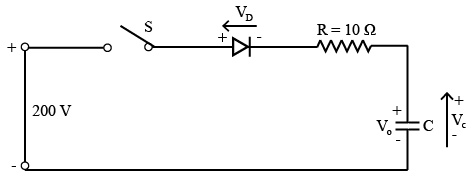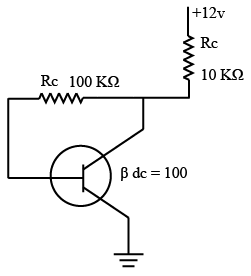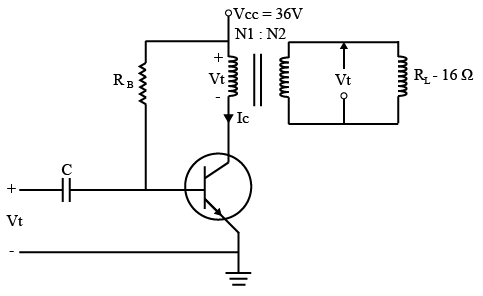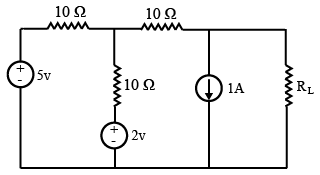# ISRO Scientist or Engineer Electrical 2015

Instructions

For the following questions answer them individually

Question 21

# A 4 bit module — 16 ripple counter uses JK F/F. If the propagation delay of each F/F is 50 nano seconds, the maximum clock frequency that can be used is equal toQuestion 22

# Figure shown below, capacitor is initially charged to $$V_o = 50 V$$ with upper plate positive. Switch S is closed at t = 0. Current through the circuit at t = 0 and final voltage across C are respectivelyQuestion 23

# The Q point value $$I_c$$ for the circuit isQuestion 24

# The frequency of oscillations of transistorized Colpitts Oscillator having tank circuit parameters $$C1 = 150 pF, C2 = 1.5 nf$$ and $$L = 50 \mu H$$ isQuestion 25

# The following distortion readings are available for a power amplifier. D2 = 0.2, D3 = 0.02 and vD4 = 0.06. The Total Harmonic Distortion (THD)isQuestion 26

# A transformer coupled class A amplifier drives a 16 Ohm loud speaker through 4:1 transformer with $$V_{cc} = 36 V$$. If the circuit delivers 2 Watts to load, the rms voltage across the load assuming transformer efficiency as 100 % isQuestion 27

# In the $$R_L$$ circuit given below, the maximum power will be transferred when value of $$R_L$$ isQuestion 28

# A-source $$V_s(t) = V \cos 100 \pi t$$ has an internal impedance of $$(4 + j3) Ω$$. If a purely resistive load is connected to this source has to extract the maximum powerout of the source, its value in Ohms should beQuestion 29

# In a transformer, exciting current is made up of two components namely magnetizing current Im and core loss current Ic, with negligible leakage impedance drop (V is supply voltage)Question 30

# The leakage flux in a transformer depends uponOR